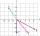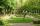# Pythagorean theorem + subtracting - math problems

#### Number of problems found: 9

• Vectors abs sum diffThe vectors a = (4,2), b = (- 2,1) are given. Calculate: a) |a+b|, b) |a|+|b|, c) |a-b|, d) |a|-|b|.
• VectorsVector a has coordinates (8; 10) and vector b has coordinates (0; 17). If the vector c = b - a, what is the magnitude of the vector c?
• WasteHow many percents are waste from a circular plate with a radius of 1 m from which we cut a square with the highest area?
• Circular ringSquare with area 16 centimeters square are inscribed circle k1 and described circle k2. Calculate the area of circular ring, which circles k1, k2 form.
• Company logoThe company logo consists of a blue circle with a radius of 4 cm, which is an inscribed white square. What is the area of the blue part of the logo?
• 6 regular polygonIt is given 6 side regular polygon whose side is 5 cm. Calculate its content area. Compare how many more cm2 (square centimeters) has a circle in which is inscribed the 6-gon.
• Two chordsIn a circle with radius r = 26 cm two parallel chords are drawn. One chord has a length t1 = 48 cm and the second has a length t2 = 20 cm, with the center lying between them. Calculate the distance of two chords.
• Central park in cityThe city park has the shape of a rectangle of 180 meters in length and 120 meters in width. People make their walk through the center of the park from one corner to the second. Calculate how many meters this way is shorter than they walked along the path
• Short cutImagine that you are going to a friend. That path has a length 120 meters. Then turn doprava and go other 630 meters and you are at a friend's. The question is how much the journey will be shorter if you go direct across the field?

We apologize, but in this category are not a lot of examples.
Do you have an interesting mathematical word problem that you can't solve it? Submit a math problem, and we can try to solve it.

We will send a solution to your e-mail address. Solved examples are also published here. Please enter the e-mail correctly and check whether you don't have a full mailbox.

Please do not submit problems from current active competitions such as Mathematical Olympiad, correspondence seminars etc...

Pythagorean theorem is the base for the right triangle calculator. Pythagorean theorem - math word problems. Subtracting Problems.